# Primavera: The Hour Planner

As we all know Primavera is an hour Planner: it calculates everything in hours. All other time units shown (days, weeks, months, years) are just a calculation.
This calculation is based on how many hours we define as a day, week, month, year.
And we define that in two different locations in Primavera:

### Option AFigure 1

On Tab: Time Periods → Hours Per Time Period: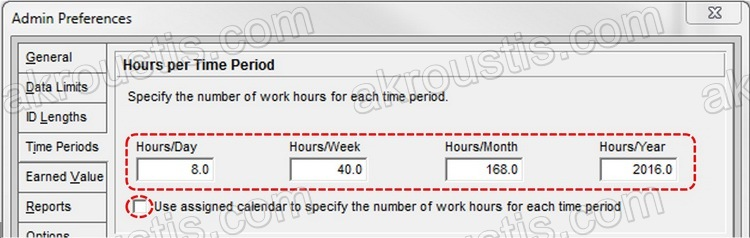Figure 2

However, these definitions are taken into account only if the item:

“Use assigned calendar to specify the number of work hours for each time period”

is unselected.

• If this item is unselected, Primavera will use these definitions for all activities in our programme. For example, based on the figure above, 1 day equals to 8 hours. For an 8-hour calendar this will be ok, but if we have also activities with 10-hour calendar this will be wrong (Primavera will show 1 day of 10-hour calendar as: 10hrs/8 = 1.25 days, see Figure 7 below). So, this “one definition for everything” is not recommended.
• If this item is selected (recommended), Primavera will use the definitions from each calendar, Option B below.

### Option B

On Tab: Time Periods → Hours Per Time Period: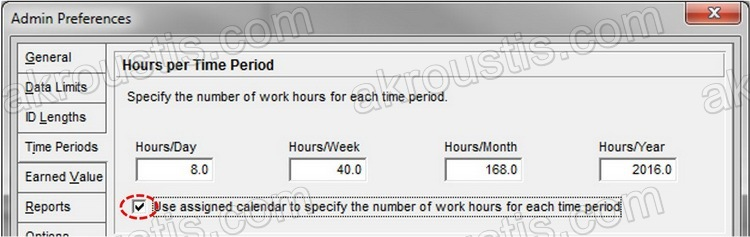Figure 3

Here, we make sure that the item:

“Use assigned calendar to specify the number of work hours for each time period”

is selected.

From the menu Enterprise → Calendars:Figure 4

On each of the Calendars if we click on button Modify: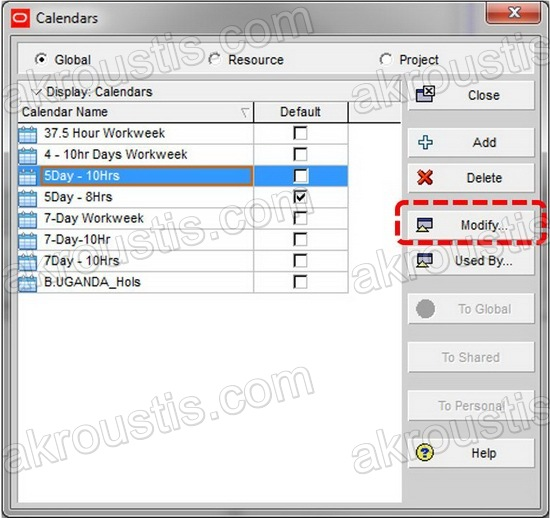Figure 5

And then click on button “Time Periods”: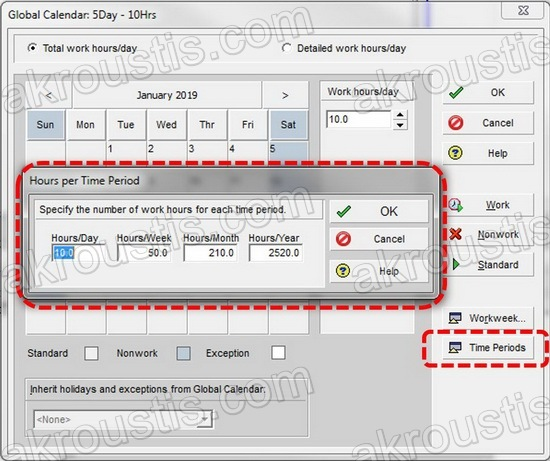Figure 6

Here we define how many hours equals one day, week etc. for each calendar separately. That means that we have different definitions of one day, week etc per calendar. That is, 1 day on an 8-hour calendar will be equal to 8 hours & 1 day on a 10-hour calendar will be equal to 10 hours. Just make sure that the definitions of what equals a day, a week etc match with all your calendars (8-hour calendar: 1 day = 8 hours, 10-hour calendar: 1 day = 10 hours and so on). Have in mind that these per calendar definitions are available only if item:

“Use assigned calendar to specify the number of work hours for each time period”

of Option A above is selected. If it is unselected, per calendar definitions are disabled (check Figure 7).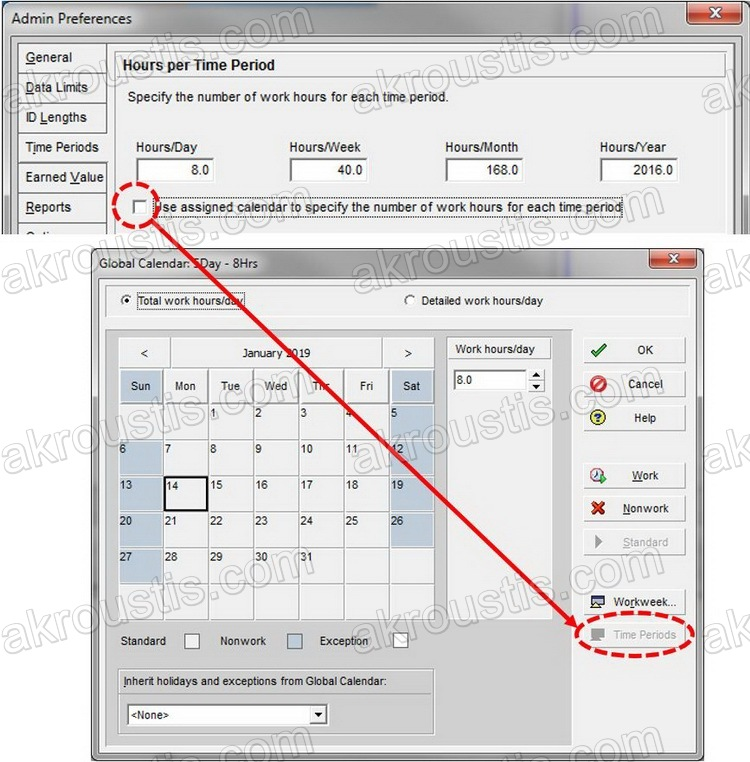Figure 7

### Case Scenarios

Based on the above information, having the item:

“Use assigned calendar to specify the number of work hours for each time period”

unselected on Option A above (Figure 2), can lead us to problems.

A. Existing Projects

For example, below we have 2 activities with different assigned calendars, an 8-Hour to the first activity and a 10-hour to the second: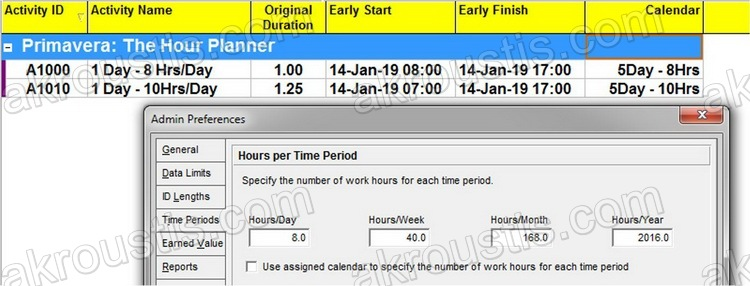Figure 8

We can clearly see that only what matches our “Global” definition of what equals a day (8 hours in our case) is displayed correctly. So Activity A1010 with 10 hours will be displayed as 10 / 8 = 1.25 Days. With the item:

“Use assigned calendar to specify the number of work hours for each time period”

enabled on Option A above the days are displayed correctly: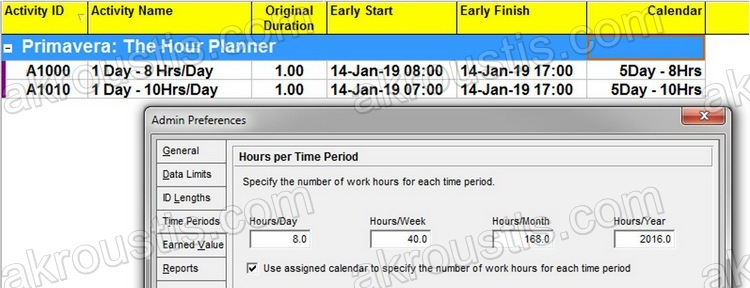Figure 9

Note: To be able to view decimals on durations you have to go to:

Edit → User Preferences → Time Units

from the menu and change Decimals to: 2, on the “Durations Format” Section (Figure 10):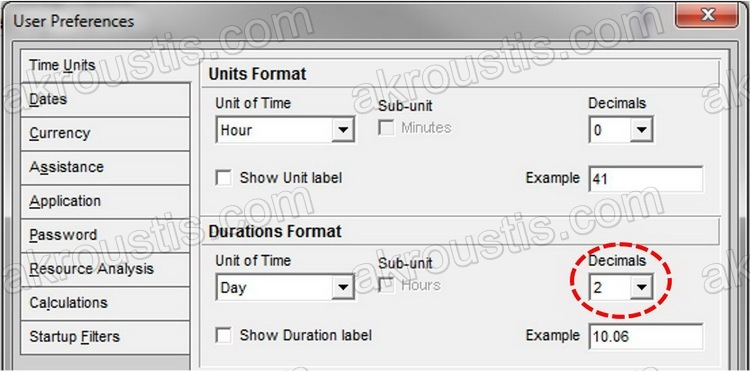Figure 10

B. Working On Projects – Changing Durations

The same translation is happening when we change durations on activities. But now Primavera will store wrong data! With the above item of Option A unselected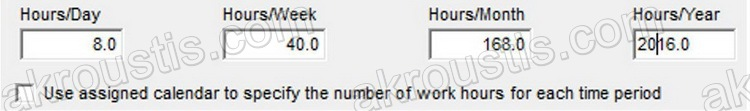and a “Global” Definition of 8 hours equals a day, having our Time Units on Durations displayed as Days (Figure 9), whenever we change an activity’s duration, Primavera will calculate:

our number of Days we entered X 8 = Total hours (Remember the title of this post?)

Below we see that if we enter 1 as duration in 10-hour Activity A1010 it will display 1.00 in Original Duration Column, but it will calculate and store:

1 X 8 =8 hours

as the activity’s duration. As a result, we see that the Activity ends 2 hours earlier: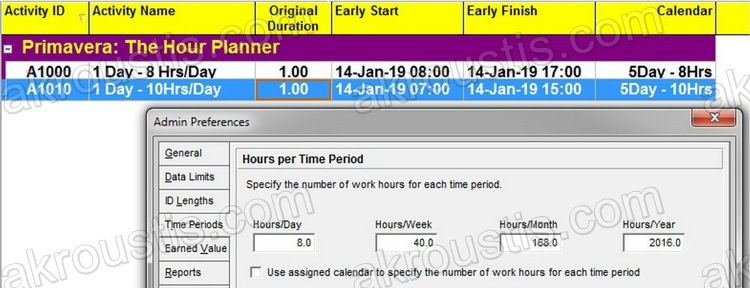Figure 11: The Activity A1010 ends at 15:00 instead of 17:00

Here Primavera stores wrong data!

Note: To display the time on start and end columns you need to go to:

Edit → User Preferences → Dates

from the menu and select: 24 Hour (13:30), and check: “Show minutes” on the “Time” Section: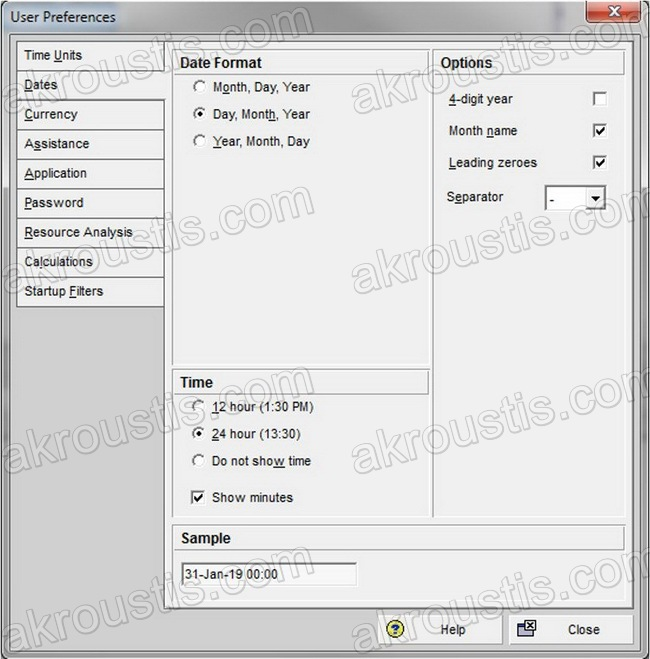Figure 12

With the above item of Option A checked Primavera will correctly calculate the hours for each activity based on each calendar’s definition of what equals a day. That is, whenever we change an activity’s duration (in days), Primavera will calculate:

our number of Days we entered X Hours/day Of Each Calendar = Total hours

Below we see that if we enter 1 as duration in 8-hour Activity A1000 it will display 1.00 in Original Duration Column, and store:

1 X 8 =8 hours

And if we enter 1 as duration in 10-hour Activity A1010 it will display 1.00 in Original Duration Column, and store:

1 X 10 = 10 hours

correctly, and now the Activity A1010 ends on the correct time (17:00):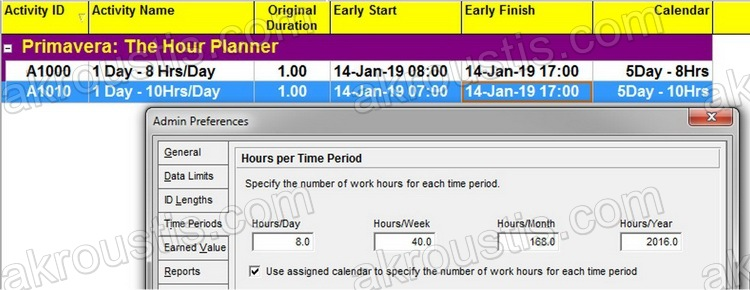Figure 13

### Conclusion

Make sure that item:

“Use assigned calendar to specify the number of work hours for each time period”

is always selected, and double check that your hours per time period definitions are correct for all your calendars (Option B above).

1.